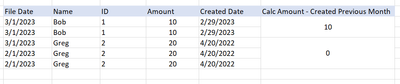cancel
Showing results for
Did you mean:Frequent Visitor

## Sum of Distinct columns by Created Previous Month Date

Hello all!

Here's the data I'm trying to calc the previous months (February) data based on these distinct columns:Thank you!!

1 ACCEPTED SOLUTIONFrequent Visitor

Thank you, gave that one a shot but wasn't quite what I was looking for. I'd been working on this seperately with this formula and got to what I needed, not sure if this is efficient?...

New Column =

CALCULATE(

SUMX(

DISTINCT (Table'[ID]), CALCULATE(MAX('Table'[Amount]))),

DATESINPERIOD('Table'[Created Date],MAX('Table'[Created Date]),-30,DAY))

3 REPLIES 3Super User

Hi @lw24

NewColumn =
VAR T1 =
CALCULATETABLE (
'Table',
ALLEXCEPT (
'Table',
'Table'[File Date],
'Table'[Name],
'Table'[ID],
'Table'[Created Date]
)
)
VAR T2 =
FILTER (
T1,
FORMAT ( 'Table'[File Date], "YYMM" ) = FORMAT ( 'Table'[Created Date], "YYMM" )
)
RETURN
MAXX ( T, 'Table'[Amount] ) + 0Frequent Visitor

Thank you, gave that one a shot but wasn't quite what I was looking for. I'd been working on this seperately with this formula and got to what I needed, not sure if this is efficient?...

New Column =

CALCULATE(

SUMX(

DISTINCT (Table'[ID]), CALCULATE(MAX('Table'[Amount]))),

DATESINPERIOD('Table'[Created Date],MAX('Table'[Created Date]),-30,DAY))Community Champion

hi @lw24

the logic is not very clear. could you elaborate it?